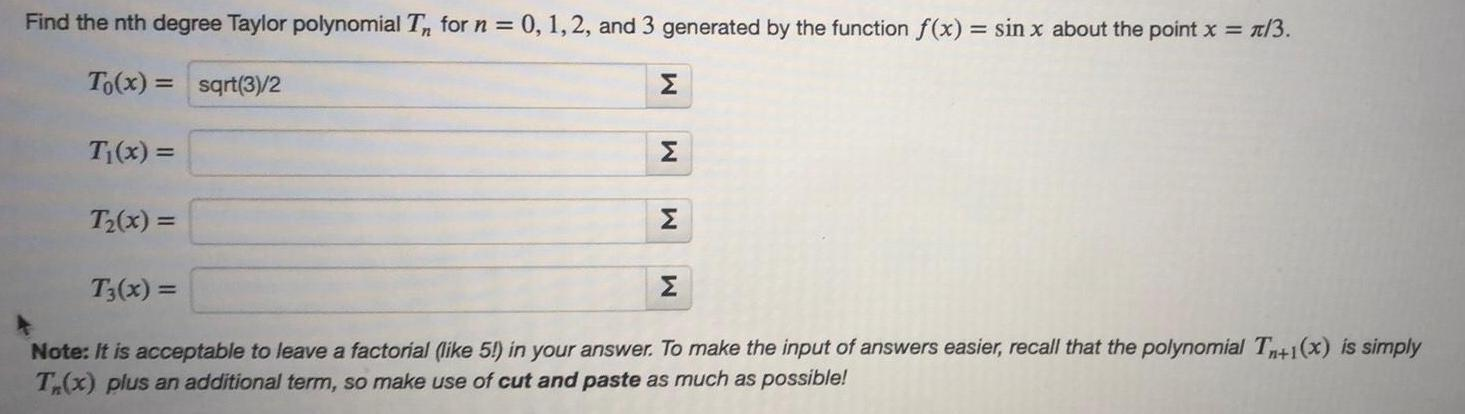Question:

# Find the nth degree Taylor polynomial Tn for n = 0, 1, 2Find the nth degree Taylor polynomial Tn for n = 0, 1, 2, and 3 generated by the function f(x) = sin x about the point x = π/3. To(x) = T₁(x) = T₂(x) = T3(x) =